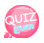# Validate Integers

Let's learn how to validate integers.
Use the filter_Var() built-in function.
And use the constant FILTER_VALIDATE_INT as argument.

## How to validate integers using filter_Var()

```filter_Var(integer,FILTER_VALIDATE_INT);
```

The first argument is an integer and the second uses the constant FILTER_VALIDATE_INT.
As shown in the example, you can change the purpose according to the value of a constant.
Returns true if the integer is valid and false.
The following example validates an integer.

```<?php
\$int = 1000;

\$checkInt = filter_Var(\$int, FILTER_VALIDATE_INT);

if(\$checkInt == true) {
echo "This is Integer";
} else {
echo "This is not Integer";
}
?>```

ResultThis time, let's test it by entering the wrong integer.

```<?php
\$int = 1000.1;

\$checkInt = filter_Var(\$int, FILTER_VALIDATE_INT);

if(\$checkInt == true) {
echo "This is Integer";
} else {
echo "This is not Integer";
}
?>```

Result